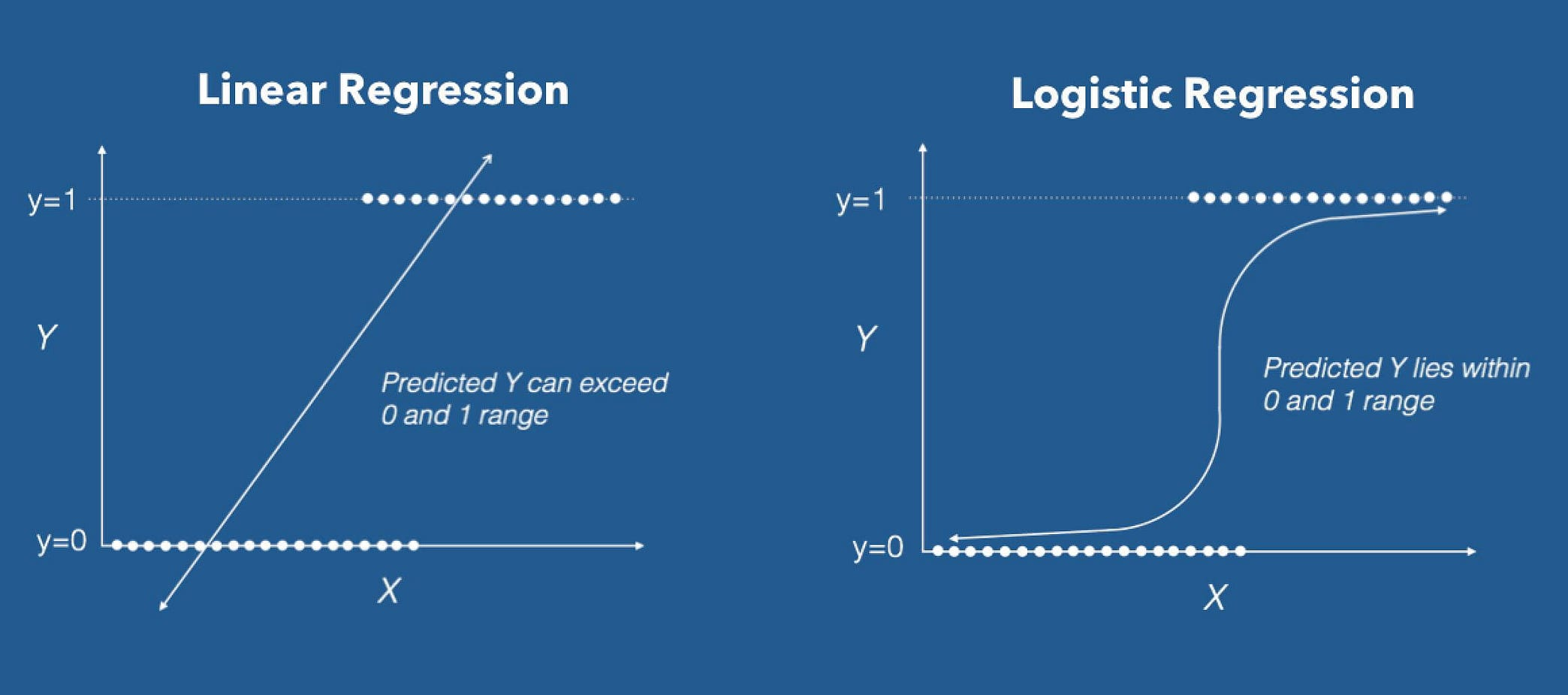By- Vineet Maheshwari
To infinity and beyond.

0 likes 0 followers ViewsVineet Maheshwari

0 Followers
Views
0 Likes

# Basic Implementation of Logistic and Linear Regression

TechnologyAs we have got some theoretical point of view on regression techniques available, now is the time to implement them using python.

Before going into the actual implementation process, we should get some information about some in-built libraries that python provide us with. These libraries make our work quite easy and time consumption is also reduced.

Like sklearn library has a module named LinearRegression and LogisticRegression. So all the internal workings are being covered up by these modules that includes calculation of cost function, gradient descent and many more things.source : machinelearningplus.com

#First of all we will import some basic libraries

import pandas as pd

import numpy as np

#now as talked earlier, we will import the modules of sklearn

from sklearn.linear_model import LinearRegression

from sklearn.linear_model import LogisticRegression

from sklearn.cross_validation import train_test_split

#train_test_split function randomly distribute array into training #and testing subsets

x = iris.data

y = iris.target

X_train, X_test, y_train, y_test = train_test_split(x, y, random_state=1)

linreg = LinearRegression() #this is to call the function

logreg = LogisticRegression()

linreg.fit(X_train, y_train) #fitting our training data

logreg.fit(X_train, y_train)

y_pred = linreg.predict(X_test)

y_pred1 = logreg.predict(X_test)

print(y_pred)

print(y_pred1)

# compute the RMSE of our predictions

print(np.sqrt(metrics.mean_squared_error(y_test, y_pred)))

So in this post we have just learnt a basic way to implement Logistic and Linear regression by using some in-built libraries. And we have used iris data set for this process.

It covers up some basic understanding regarding Logistic and Linear regression implementation.